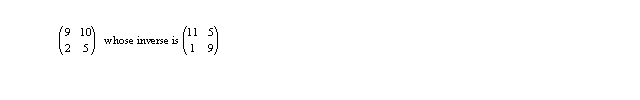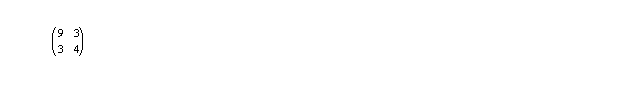Project #3 Cryptography

Introduction

In this project, we introduce the reader to some of the fundamental notions of crytography in a linear algebraic context. Cryptography has long been an important issue in the realm of computers, mainly due to security needed for passwords. In recent times, due to the Internet, it has taken on more importance with sensitive information of all kinds, such as credit card numbers, passing over media which are fairly easy to monitor by unintended third parties.

In most systems, a message sent is encoded according to some algorithm, sent to its destination, and decoded according to the inverse of the initial algorithm. Before doing this, characters need to have a numerical equivalent. There are, of course, many ways of doing this. One could simply have A thru Z represented by the integers 1-26. If small letters were desired, they could be 27-52. Or one could use the ASCII system (American Standardized Code for Information Interchange), in use on most computers whenever a "text" file is created.

In what follows, we simplify matters by only using capital letters, A-Z and a character for a space. We will equate these with the 2 digit numbers 0-26; "LINEAR ALGEBRA" is equated to the number 1209140501180001120705021801 (for example, the E is represented by 05, the space by 00 etc).

Modulo Arithmetic and Zn

To keep things organized, we will associate all 2 digit integers with characters A-Z and space. The first 26 integers are easy; we have already done that. But what character do we associate 87 with? This is taken care of the modulo approach for integers.

Two integers are said to be equivalent, modulo 27, if they differ by an integral multiple of 27, or, mathematically,

x eq. y       <=>       x - y = 27k        for some integer k

Thus 3 and 30 are equivalent, 8 and 35, -6 and 21 etc. We end up putting all integers into 27 different "classes", 0,1,2,3,...,26. The class "3" stands for {. . . , -24,3,30,57,. . .}, while "0" stands for {. . . ,-27,0,27,54,. . . }. "21" and "73" are not equivalent because their difference, 52, is not a multiple of 27.

Addition, subtraction and multiplication are easy. To multiply 9 time 8, the answer is the class that 72 falls into, 18 in this case (18 = 72 - 2*27). Other simple examples are:

9+ 16 = 25 and 18 + 9 = 0. This last example means that 9 is additive inverse of 18.

Fractions in the usual sense (such as 1/4) do not exist. However, if we recall the reason for 1/4 existing, it was as the multiplicative inverse of 4, or the number which when multiplied by 4 gave us 1 (the multiplicative identity).

Let us suppose we want the multiplicative inverse of 5. This means we wish to solve the algebraic equation 5x = 1, or, in words, to find a number in {0,1,2,. . . ,26} which, when multiplied by 5 gives us 1. The solution is x=11 since 5*11 = 55 = 2*27 +1=1.

Exercise: set up addition and multiplication tables for Z27. Note that since both addition and multiplication are commutative (x + y = y+ x and x*y = y*x), your work may be cut in half in each case.

Do all nonzero numbers have multiplicative inverses? What implications does your answer have for the suitability of the shift algorithm?

A Simple Encoding Scheme

Once the message has been converted to a string of 2 digit integers, we want to encode it. We can take each integer and add a fixed integer to it. The resulting string is then transmitted and the receiver subtracts the same integer to recover the message. Suppose our fixed integer is 18. Then the string for LINEAR ALGEBRA would be converted

from:        1209140501180001120705021801

to             0300052319091819032523200919

(noting that we have replaced any sum greater than 27 with its equivalent, modulo 27). The receiver would subtract 18 from each 2 digit number, replacing any negative results with their equivalent from 0 to 26. Try it! This approach is called a shift algorithm. The number 18 is the key of the algorithm.

Security Considerations

Designers of encryption algorithms consider worst case scenarios. This often amounts to assuming a 3rd party has gotten a message in both encoded and decoded form. The question is: given this, can he recreate the algorithm and thus convert all future messages?

If this is theoretically possible, then two questions result: how much effort would it take him and how much information (length of message) would he need to do it? What would be your analysis of the shift algorithm described above?

The Hill Cipher

Lester Hill, in 1929, published a scheme using some of the above ideas as well as some linear algebra. Its essence is presented here. It is far from state of the art, but experience with it helps one understand the basic issues involved with cryptography.

Suppose we decide to encode numbers two at a time rather than one. If x and y are two 2 digit numbers, each representing a letter or space as before, and one constructs the 2x1 column d=(x y)t ("d" for decoded) ,then we take a 2x2 matrix A, whose entries are from Z27 and form the product Ad = e (where "e" is for encoded). To recover the original message, we simply compute d = A-1e. This would be repeated for all of the pairs of numbers making up the original message.

For example, if we are using the same message ("LINEAR ALGEBRA") then we would start by encoding the column (12 09)t for LI. We need a matrix - its requirements are that its entries be from Z27and it is invertible. A suitable matrix would beso that multiplying (12 09)t by A yields (9 15)t , the encoded pair, IO.

Computational Questions

At this point, one needs to be able to decide if a 2x2 matrix of integers from Z27 is invertible. Decide on a criteria.

Next one needs to be able to actually compute inverses. All results must have entries which are integers from Z27. As always, the acid test is: does the product of the proposed inverse and the original matrix give I2, the identity matrix? Remember, if a multiplicative inverse of a number is needed, the multiplication table developed earlier may help!

Make up your own matrix A, encode LINEAR, and then use A-1 to decode your result.

Is it possible for a 2x2 matrix to be invertible in R (the real numbers) but not invertible in Z27 ?

Mathematical Questions

Why do we not want to use a singular matrix for A? If the entries in A are from Z27 then there are 274 possible matrices to choose from. Write a program using your software package to see how many of these are singular (using whatever your criteria for invertibility was above). What percentage of such 2x2 matrices are invertible? Consider for example the matrixThe number of invertible matrices is important - not only does it give use more to chose from but it makes the task of the 3rd party harder in terms of effort needed to "guess" at it.

How do the all of the above answers change if we encode 3 characters at a time instead of 2?

Now suppose the 3rd party is to gain some information in both encoded and decoded form. How much would he need to be able to reconstruct A? If he were to know that the letters NE encoded to BG would that be enough to reconstruct A?

Suppose we find the results using a single 2x2 matrix to encode two characters at a time unacceptable. How would you compare the following two alternatives: encode 3 characters at a time or use a pair of invertible 2x2 matrices to encode alternate pairs of characters (one for the first pair, the other for the second pair, etc etc)?

References

L.S. Hill Cryptography in an algebraic alphabet. American Mathematical Monthly, 36 (1929), 306-312.